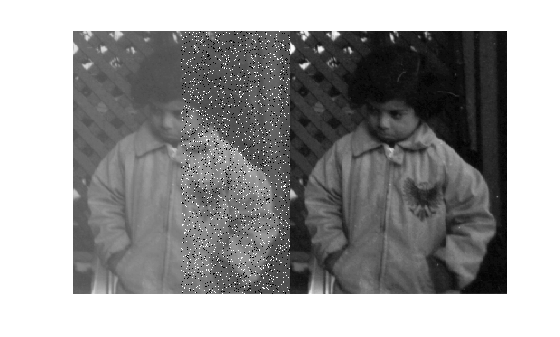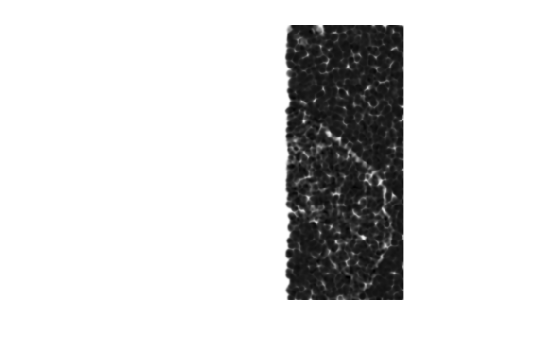# Obtain Local Structural Similarity Index

This example shows how to measure the quality of regions of an image when compared with a reference image. The `ssim` function calculates the structural similarity index for each pixel in an image, based on its relationship to other pixels in an 11-by-11 neighborhood. The function returns this information in an image that is the same size as the image whose quality is being measured. This local, pixel-by-pixel, quality index can be viewed as an image, with proper scaling.

Read an image to use as the reference image.

`ref = imread('pout.tif');`

Create an image whose quality is to be measured, by making a copy of the reference image and adding noise. To illustrate local similarity, isolate the noise to half of the image. Display the reference image and the noisy image side-by-side.

```A = ref; A(:,ceil(end/2):end) = imnoise(ref(:,ceil(end/2):end),'salt & pepper', 0.1); figure, imshowpair(A,ref,'montage')```Calculate the local Structural Similarity Index for the modified image (A), when compared to the reference image (ref). Visualize the local structural similarity index. Note how left side of the image, which is identical to the reference image displays as white because all the local structural similarity values are 1.

```[global_sim local_sim] = ssim(A,ref); figure, imshow(local_sim,[])```